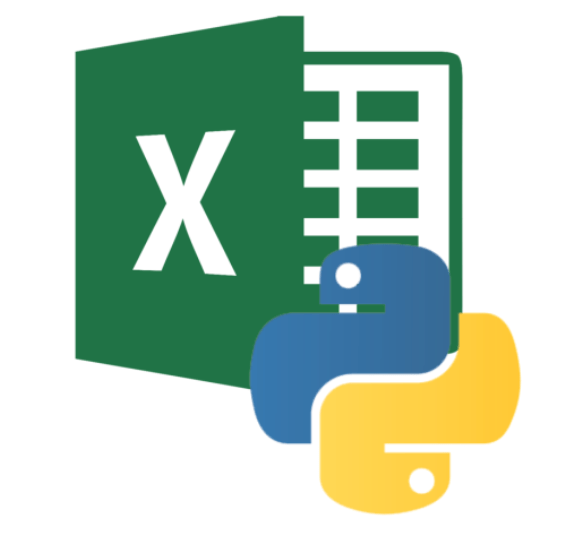# Python – Reading an Excel file using xlrdHello Friends,

Let’s continue exploring Python

Today in this article we are going to discuss about how to install xlrd in Python using – pip and reading excel file using xlrd

What is xlrd?

xlrd is a module that allows Python to read data from Excel files.

### Install XLRD using pip in Python

If we want to use python to read the data from the Excel table, we need to install xlrd package in Python

To Install xlrd package follow the steps given-

1. Open command prompt
2. If we want to use pip to install the xlrd package then we can do as follows:
``pip install xlrd``

We can also install xlrd package using easy install follows:

``easy_install xlrd``

We could uninstall xlrd using “uninstall” attribute of pip

``pip uninstall xlrd``

How to read Excel file in the Python program

• Import the module – xlrd
``import xlrd``
• We have to give the location of the file where our file is stored
``````location = "D:\Python programs\Excelfiletest.xls"
``````
• xlrd has the function – open_workbook() which takes the parameter – path of excel file and opens the workbook, returns the workbook object as
``````
workbook = xlrd.open_workbook(location)``````
• Once we have the workbook object we can read the any sheet using workbook.sheet _by_index()
• workbook.sheet _by_index() – takes the parameter sheet index, here we are reading first sheet from the workbook
``sheet = workbook.sheet_by_index(0)``
• Now we can read the cell value using sheet.cell_value()

Complete program :

``````import xlrd

location = "D:\Python programs\Excelfiletest.xls"

workbook = xlrd.open_workbook(location)
sheet = workbook.sheet_by_index(0)

print(sheet.cell_value(0, 0))``````

xlsx file is not supported in xlrd module

1. xlsx file is not supported in xlrd module
2. For example, if we try to read xlsx file it will show the error – xlrd.biffh.XLRDError: Excel xlsx file; not supported

### 4 Responses

1. August 22, 2021

[…] previous article – Python – Reading an Excel file using xlrd we have discussed about how to read the single […]

2. March 6, 2022

[…] To get the solution check this link’s – part 1 – https://knowledge-junction.com/2021/08/22/python-how-to-read-the-complete-excel-file-using-xlrd/ […]

3. March 14, 2022